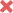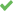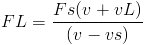Doppler effect

Homework Statement

:
An ambulance has a siren of frequency 880 Hz. You are sitting at a stop light as the ambulance passes you, traveling at a constant 22 m/s. If the speed of sound is 343 m/s, what is the change in the frequency that you hear as the ambulance passes you?

• A

-53 Hz

•B

-60 Hz

• C

-56 Hz

•D

-113 Hz

• E

-116 Hz
the correct Answer is D : -113 Hz

Homework EquationsWHERE FL and vL are the frequency and velocity of sound heard by the personL
fs and vs are the frequency and velocity of sound emmited by the source

The Attempt at a Solution

using the equation we get FL=880x(343+0)/(343-22) therefore FL=940.3Hz
the difference is FL-Fs=940.3-880=60.311Hz
but its not correct.
the correct answer is -113Hz why???